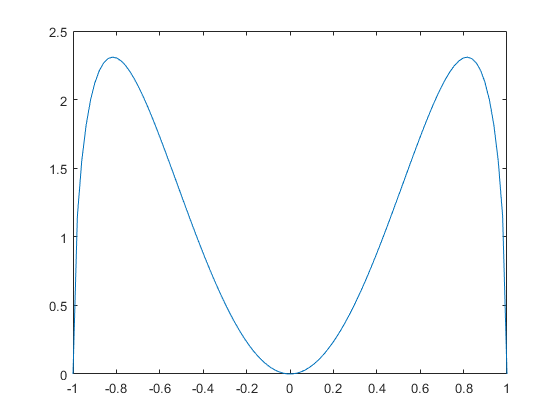Hi, rooters,

I want to fit a 1d histogram by using TF1 g(x).
But the g(x) is obtained by integral variable y for f(x,y) based on some physical process.

And The integration process is numerical integration. (The analytic integral is too complex to work out by Mathematica software)

I want to how to define my fit function, could you please provide an example for me?

Best regards,
hurricane.

There is a section in the Manual explaining how to proceed.

What I want to know is how to obtain my fit function TF1 by integral TF2.

In the fit function you define, you can use any complex C++ programming or access the data you need. Have you checked the ROOT Math libraries to see if some tools there answer your question ? And, at the end, if no predefined tools are satisfactory for you, you can program yourself the algorithm you need. May be @moneta has some ideas.

Hi,
There exists many example for this. A recent and simple one is this:

Thank you very much .

Best regards,
hurricane

Thank you very much!
The function Integral() may be helpful I guess.

Best regards,
hurricane

My fit function is g(x), which obtained by integral f(x,y) from y = -sqrt(1-x^2) to y = sqrt(1-x^2).
And the f(x,y) = 3x^2+2y.

``````#include "TF2.h"
#include "TH2.h"
#include "TCutG.h"
#include "TMath.h"
#include "TCanvas.h"
#include "TStyle.h"

Double_t f2(Double_t *x, Double_t *par) {
return par*(3*x*x+2*x);
}

Double_t gx(Double_t *x, Double_t *par) {
Double_t yrange = {-TMath::Sqrt(1-x*x),TMath::Sqrt(1-x*x)};
return  f2->Integral(yrange,yrange);
}

TCanvas *fit2a() {
TCanvas *c = new TCanvas();
gStyle->SetOptStat(kTRUE);
gStyle->SetPalette(57);
const Int_t npar = 1;
Double_t f2params[npar] = {2};
auto f1 = new TF1("f1",gx,-1,1, npar);
f1->SetParameters(f2params);

//Create an histogram and fill it randomly with f2
auto h1 = new TH1F("h1","From f1",40,-1,1);
Int_t nentries = 100000;
h1->FillRandom("f1",nentries);
f1->SetParameters(f2params);
h1->Fit("f1","N");
h1->Draw();
f1->Draw("same");
return c;
}

``````

But the result is:

Best regards,
hurricane

Hi,
You did not follow closely the example above. You need to create an internal TF1 object to define the f2(y) function. You can create for every call of gx(x) as in the example or , in case it is too time consuming, create as a global object or use a functor class and create in its constructor (cleaner and more efficient solution).
I attach below the case where the TF1 object is created inside g(x) as in the cited example.

``````#include "TF2.h"
#include "TH2.h"
#include "TCutG.h"
#include "TMath.h"
#include "TCanvas.h"
#include "TStyle.h"

Double_t f2(Double_t *x, Double_t *par) {
return par*(3*x*x+2*x);
}

Double_t gx(Double_t *x, Double_t *par) {
Double_t yrange = {-TMath::Sqrt(1-x*x),TMath::Sqrt(1-x*x)};
TF1 func2("f2",f2,TMath::Infinity(),-TMath::Infinity(), 1);
func2.SetParameter(0, par);
return  func2.Integral(yrange,yrange);
}

TCanvas *fit2a() {
TCanvas *c = new TCanvas();
gStyle->SetOptStat(kTRUE);
gStyle->SetPalette(57);
const Int_t npar = 1;
Double_t f2params[npar] = {2};
auto f1 = new TF1("f1",gx,-1,1, npar);
f1->SetParameters(f2params);

//Create an histogram and fill it randomly with f2
auto h1 = new TH1F("h1","From f1",40,-1,1);
Int_t nentries = 100000;
h1->FillRandom("f1",nentries);
f1->SetParameters(f2params);
h1->Fit("f1","N");
h1->Draw();
f1->Draw("same");
return c;
}
``````

Cheers

Lorenzo

The result of this code is:

But the g(x) = 6x.^2sqrt(1-xx), the right plot is:So the integral process in ROOT may be wrong, but I can’t find the bug…

Best regards,
hurricane

Hi,
you are right, the code above is wrong, since you want to compute an integral of f(x,y) in dy. You need in that case to define x as a parameter for ‘f2’. .Here is the corrected code:

``````#include "TF2.h"
#include "TH2.h"
#include "TCutG.h"
#include "TMath.h"
#include "TCanvas.h"
#include "TStyle.h"

Double_t f2(Double_t *x, Double_t *par) {
return par*(3*par*par+2*x);
}

Double_t gx(Double_t *x, Double_t *par) {
Double_t yrange = {-TMath::Sqrt(1-x*x),TMath::Sqrt(1-x*x)};
TF1 func2("f2",f2,TMath::Infinity(),-TMath::Infinity(), 2);
func2.SetParameter(0, par);
func2.SetParameter(1, x);
return  func2.Integral(yrange,yrange);
}

TCanvas *fit2a() {
TCanvas *c = new TCanvas();
gStyle->SetOptStat(kTRUE);
gStyle->SetPalette(57);
const Int_t npar = 1;
Double_t f2params[npar] = {2.};
auto f1 = new TF1("f1",gx,-1,1, npar);
f1->SetParameters(f2params);

//Create an histogram and fill it randomly with f2
auto h1 = new TH1F("h1","From f1",40,-1,1);
Int_t nentries = 100000;
h1->FillRandom("f1",nentries);
f1->SetParameters(f2params);
ROOT::Math::IntegratorOneDimOptions::SetDefaultIntegrator("Gauss");
h1->Fit("f1","N");
h1->Draw();
f1->Draw("same");
return c;
}
``````

Thank you very much .
The code works well and solved my problem.

Best regards,
hurricane

This topic was automatically closed 14 days after the last reply. New replies are no longer allowed.